# Oriented cohomology

• ## General Introduction

Examples include algebraic cobordism, algebraic K-theory, and motivic cohomology.

<]]>

<]]>
• ## Online References

See references under Algebraic cobordism. The main reference is the book by Levine and Morel

Push-forwards in oriented cohomology theories of algebraic varieties, by Ivan Panin and Alexander Smirnov: K0459. A sequel is in Panin

<]]>

<]]>

<]]>
• ## Properties

(Following Levine-Morel p. 14) Fix a base scheme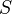. Write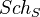for separated schemes of finite type over. Write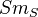for the full subcat of smooth quasiprojective-schemes. A full subcat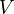ofis admissible if it satisfies: 1. It containsand the empty scheme. 2. If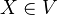and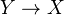is quasi-projective, then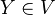. 3. It is closed under products and disjoint unions.

Definition of transverse morphisms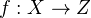and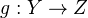. Def of additive functor fromto category of commutative, graded rings with unit: a contravariant functor taking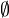to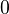and disjoint unions to products.

Letbe admissible. An oriented cohomology theory onis given by:

1. An additive functor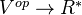2. For each projective morphism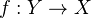inof relative codimension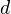, a HM of graded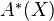-modules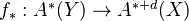satisfying the following:

1. Reasonable behaviour of push-forward wrt identity and compositions.
2. For a pullback square given by two transverse morphism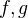, with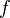projective of relative dimension, one has the commutation relation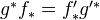.
3. (PB) Let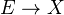be a rank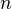VB over some. Then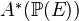is a free-module, with a certain explicit basis.
4. (EH) Letbe a VB over someand let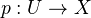be an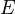-torsor. Then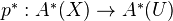is an isomorphism.

The abbreviations for points 3 and 4 stand for Projective Bundle formula and Extended Homotopy property.

Now suppose the base scheme is a field. Can use Grothendieck's method to define Chern classes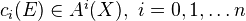, for a rankvector bundle. The first Chern class of a tensor product of line bundles is given by a commutative formal group law over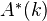.

Example: The Chow ring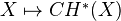is an oriented cohomology theory on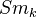. We have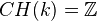and the formal group law is the additive FGL.

Example: The Grothendieck group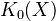of locally free coherent sheaves is a ring with multiplication induced from tensor product. The functor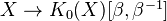is an oriented cohomology theory. The group law is the multiplicative FGL: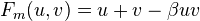.

In characteristic zero, the Chow ring functor is the universal ordinary OCT on. A rational analogue holds over any field. Examples of ordinary cohomology theories: l-adic cohomology, de Rham cohomology over a field of char zero, the even part of Betti cohomology associated to a complex embedding of the base field. In some sense the universality of the Chow ring explains the cycle class map in all these theories.

Over any field, the K-group functor described above is the universal multiplicative and periodic OCT on.

Theorem: Assume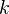has characteristic zero. Then there exists a universal oriented cohomology theory on, denoted by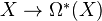, which we call algebraic cobordism. This universality means what you think it means.

Levine-Morel claims (p. 24) that any oriented bigraded theory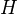(Bloch-Ogus???) gives an oriented theory, by the formula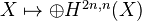.

<]]>

<]]>
• ## Open Problems

In characteristic zero, the Chow ring functor is the universal ordinary OCT on. Conjecture: This holds over any field.

Would it make sense to define algebraic cobordism over a field of char zero? Is resolution of singularities the problem?

<]]>

<]]>
• ## Computations and Examples

Oriented Cohomology and Motivic Decompositions of Relative Cellular Spaces , by Alexander Nenashev and Kirill Zainoulline

<]]>

<]]>
• ## Some Research Articles

Panin and Yagunov on Poincaré duality

Something interesting by Merkurjev

Panin on Riemann-Roch: K0552

Nenashev on Gysin maps

Borel-Moore Functors and Algebraic Oriented Theories , by Mona Mocanasu

<]]>

<]]>